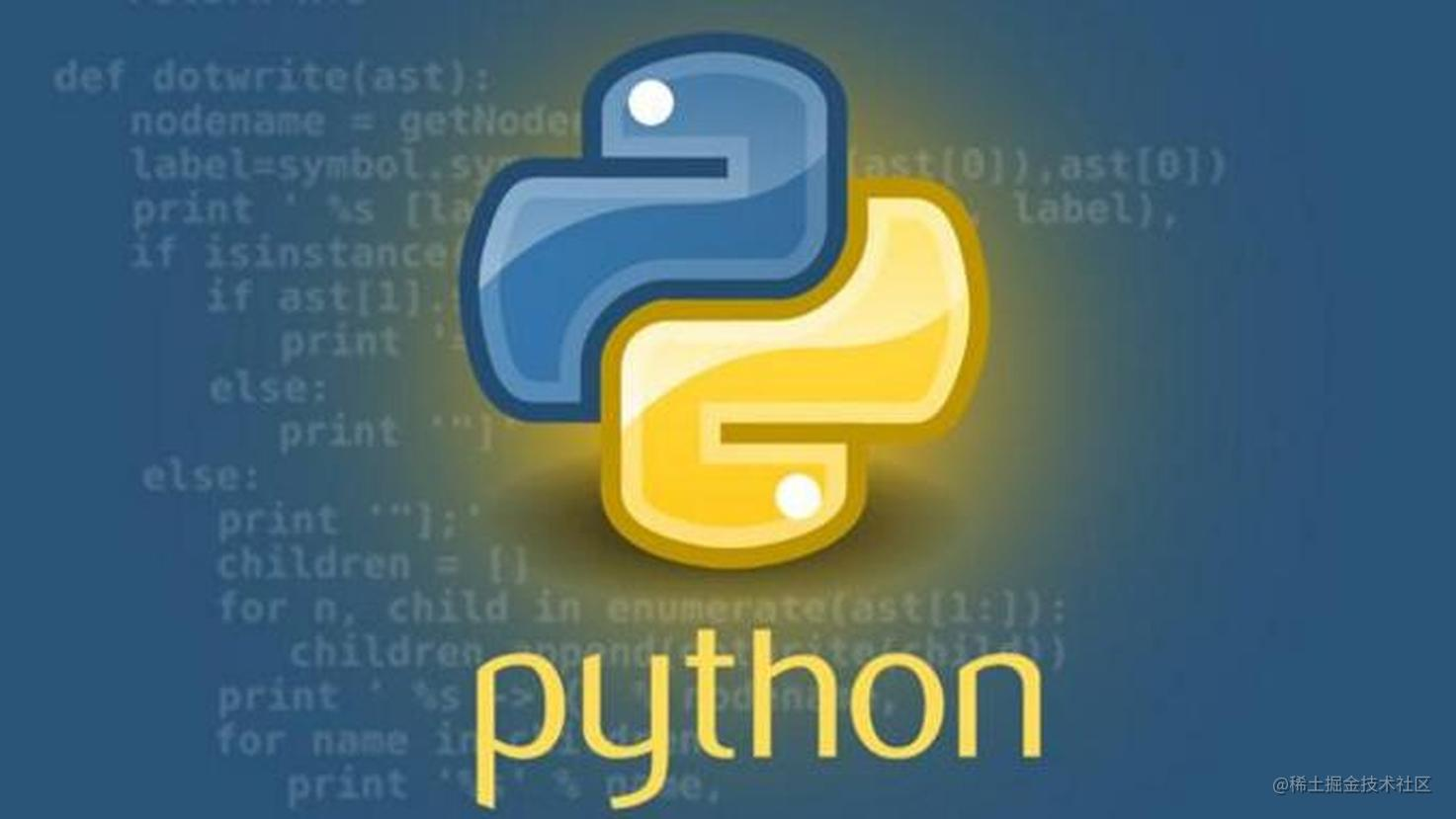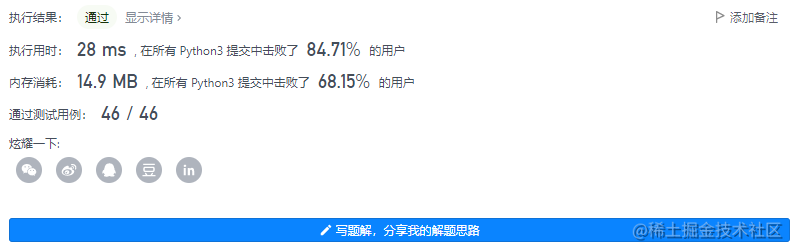# leetcode_157 用 Read4 读取 N 个字符### 要求

API read4 可以从文件中读取 4 个连续的字符，并且将它们写入缓存数组 buf 中。

``````参数类型: char[] buf4

``````File file("abcde"); // 文件名为 "abcde"， 初始文件指针 (fp) 指向 'a'
char[] buf4 = new char; // 创建一个缓存区使其能容纳足够的字符

``````参数类型:   char[] buf, int n

``````输入： file = "abc", n = 4

``````输入： file = "abcde", n = 5

``````输入： file = "abcdABCD1234", n = 12

``````输入： file = "leetcode", n = 5

• 你可以假定目标缓存数组 buf 保证有足够的空间存下 n 个字符。

### 核心代码

``````"""

@param buf4, a list of characters
@return an integer

# Below is an example of how the read4 API can be called.
file = File("abcdefghijk") # File is "abcdefghijk", initially file pointer (fp) points to 'a'
buf4 = [' '] * 4 # Create buffer with enough space to store characters
read4(buf4) # read4 returns 4. Now buf = ['a','b','c','d'], fp points to 'e'
read4(buf4) # read4 returns 4. Now buf = ['e','f','g','h'], fp points to 'i'
read4(buf4) # read4 returns 3. Now buf = ['i','j','k',...], fp points to end of file
"""

class Solution:
"""
:type buf: Destination buffer (List[str])
:type n: Number of characters to read (int)
:rtype: The number of actual characters read (int)
"""
tmp = ["","","",""]
cnt = 0
while tmp != ["","","",""]:
for i in range(4):
if tmp[i]:
buf[cnt] = tmp[i]
cnt += 1
if cnt == n + 1:
return n
tmp = ["","","",""]